# Percentage Increase and Decrease Word Problems

Percentage Increase and Decrease Word Problems• To find 10%, divide a number by 10.
• The original mass of chocolate is 200 grams.
• 200 ÷ 10 = 10 and so 10% of 200 grams in 20 grams.
• To increase an amount by 10%, add 10% to the original amount.
• 200 + 20 = 220. Therefore the new mass is 220 grams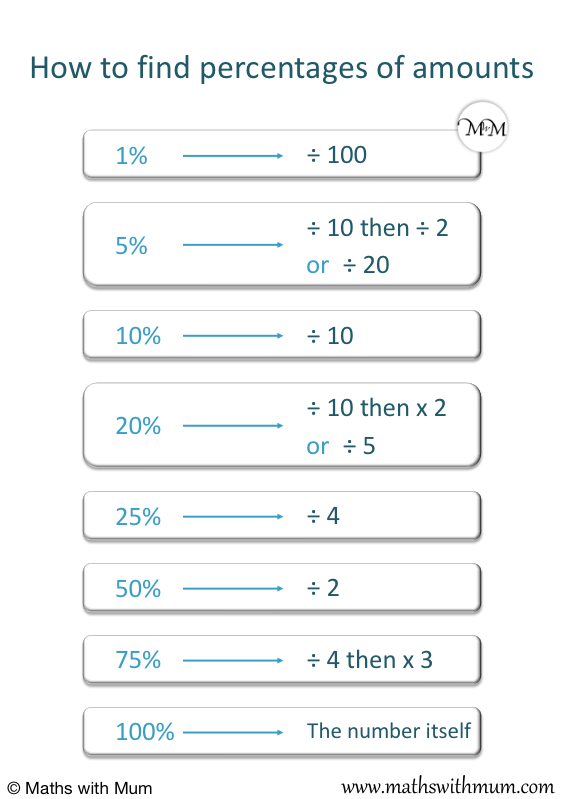Add the percentage to the original amount to increase by a percentage.

Subtract the percentage from the original amount to decrease by a percentage.• To find 40%, first find 10% and then multiply it by 4.
• 10% is found by dividing the number by 10. £50 ÷ 10 = £5 and so, 10% is £5.
• We multiply 10% by 4 to get 40%. £5 × 4 = £20 and so, 40% is £20.
• In a sale, the price is decreased.
• To decrease by a percentage, subtract the percentage from the original number.
• £50 – £20 = £30 and so, the new price is £30.Supporting Lessons

# Percentage Change Word Problems

## How to Work out Percentage Change

To work out percentage change, follow these steps:

1. Work out the percentage by dividing the original number by 100 and multiplying by the percentage.
2. For a percentage increase, add this percentage to the original number.
3. For a percentage decrease, subtract this percentage from the original number.

For example, in this percentage change question, we will increase $52 by 23%. Step 1 is to find the percentage. To find 23%, divide$52 by 100 and then multiply by 23.$52 ÷ 100 × 23 =$11.96. Therefore 23% of $52 is$11.96

We are going to increase the value by 23%.

Step 2 is to add the percentage to the original number.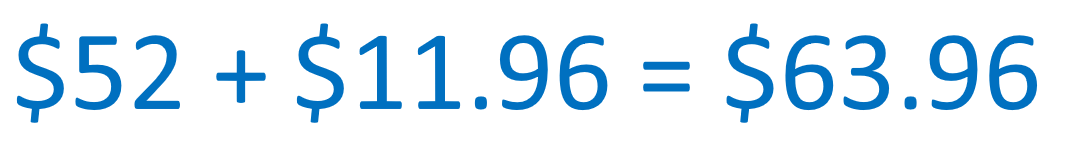$52 +$11.96 = $63.96. Therefore$52 increased by 23% is $63.96 Here is another example with percentage decrease. We will decrease$75 by 36%.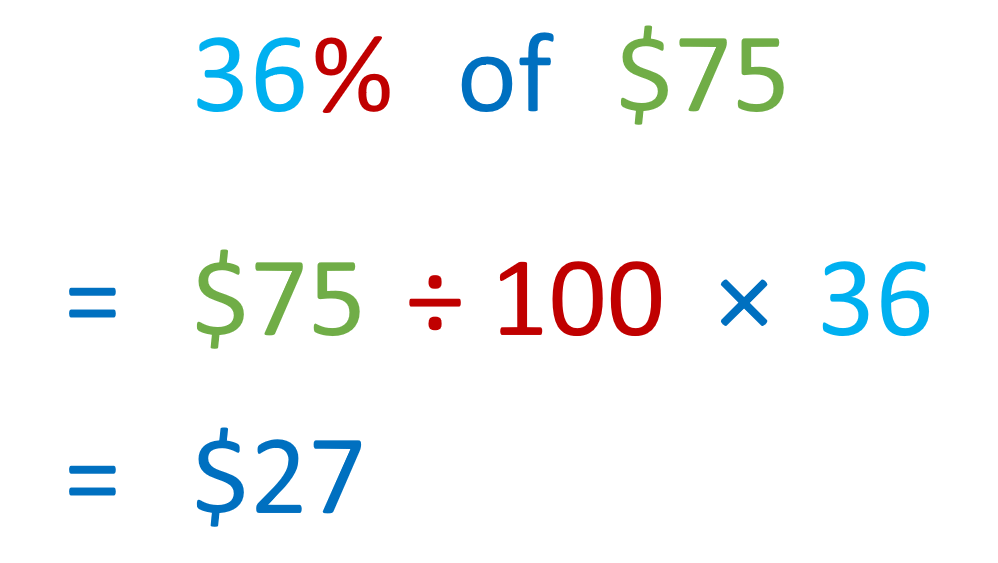The first step is to find the percentage by dividing the amount by 100 and multiplying by the percentage.

$75 ÷ 100 × 36 = 27. Therefore 36% of$75 is \$27.

This is a percentage decrease question.

The next step is to subtract the percentage from the original amount.Here are some common percentages that can be found without a calculator:

• To find 1%, divide by 100.
• To find 5%, divide by 20.
• To find 10%, divide by 10.
• To find 20%, divide by 5.
• To find 25%, divide by 4.
• To find 50%, divide by 2.

## Percentage Increase Word Problems

Here is a real life example of word problems involving percentage increase.In this example, we increase the mass of a bar of chocolate by 10%The first step is to work out the percentage value. We want to find 10%.

To find 10% of a number, divide it by 10.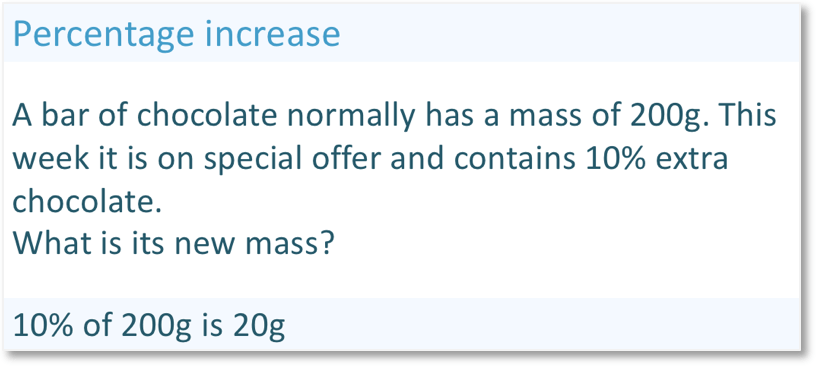10% of 200 grams is 20 grams.

This is a percentage increase word problem, so we add the percentage to the initial amount.200 + 20 = 220 and so, the new mass of chocolate is 220 g.

## Percentage Decrease Word Problems

Here is a real life example of word problems involving percentage decrease.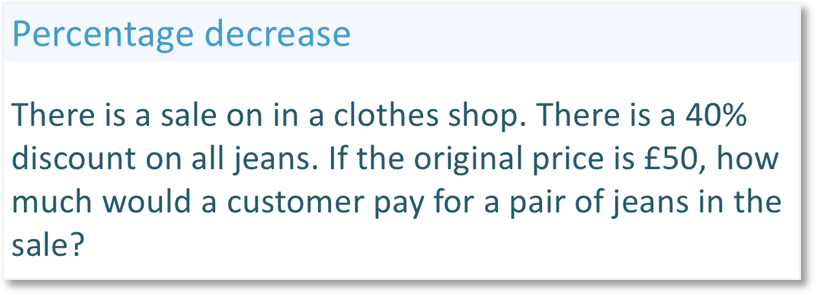This is a percentage decrease problem because we want to find the new price of the item after it has gone on sale. A sale means that the price has gone down.

The first step is to find the percentage value.

40% is found by finding 10% and multiplying this by 4.

10% is £5 because £50 divided by 10 = £5.We multiply 10% by 4 to find 40%. 40% is £20.This is a percentage decrease question because we want to take 40% off the total price.

In a percentage decrease problem, subtract the percentage from the original amount.

£50 – £20 = £30 and so the sale price is £30.Now try our lesson on Converting Percentages to Fractions where we learn how to write a percentage as a fraction.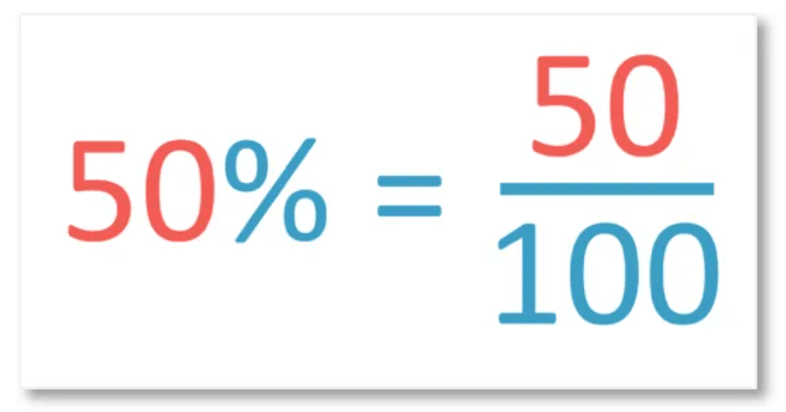error: Content is protected !!